###### MAE 6226, 5/5/2015, the George Washington University. Written by George Teel¶Hello everyone!

This project has been inspired by an absolutely beautiful aircraft, known as the Caspian Seamonster (KM). The origins of this specific type of craft was a military sea plane that the United States of America (USA) discovered being built during the cold war by the Union of Soviet Socialist Republics (USSR) in 1966. This Ekranoplan has a few variants with the first few being military versions known as the Lun Class, having antiship missiles attached to it. A plane designed for invasion, quick agile transportation, and efficiency for military use, the ekranoplan was Russia's answer to their military needs. But as we all know, the cold war was an arms race, and many technologies such as this one were cancelled and mostly forgotten to rot away, the pilots included sadly. This project more specficially will be a case study of the A-90 Orlyonok (Орлёнок), which was designed to be a modern non militarized transport vehicle. These beautiful crafts are technological wonders as they are a combination of both sea technologies and aerospace. An intense study of of materials, structural engineering, airfoil design, Wing in Ground effect, and engines in salt water environments all designed to create these amazing constructs. Here's a quick video of the military "Caspian Seamonster" in action, to pique some interest!

In :
from IPython.display import YouTubeVideo

Out:

And if you want some good background music, I have provided a link at the very bottom of the page or just click here! Feel free to read and listen if you want for some good atmosphere, I highly suggest it.## The Concept¶

The fundamental design behind the ekranoplan is utilizing ground effect.

"What is ground effect" you might be asking? It's really a simple concept, or atleast can be simplified. Imagining the fluid flow around an airfoil, vortices get created, and the wind wake is formed behind the wings. But, when one flies close to the ground, these flows get disrupted which change the induced drag.

Taken from Federation of American Scientists website: "Wing-In-Ground (WIG) effect craft take advantage the fact that the aerodynamic efficiency of a wing, and particularly its lifting capacity, improves dramatically when is operated within approximately one-half of its span above ground or water, in what is termed ground effect."

Taken from a Google translated page of a russian paper: "lift can reach 50%, the increase aerodynamic efficiency (the ratio of lift to the drag force) - 1, 5 ~ 2, 5 or more times. Influence of the screen on the wing - a very complex physical phenomenon, and full of clarity and understanding the mechanism of this effect yet."

Another conceptual image is an air cushion that gets formed under the wings which makes crafts fly longer or "hover" over the ground due to the pressure differential. This affects all flying crafts (and birds too!) and can cause variations (potentially problems) while landing. In this case, these Wing-In-Ground Effect (WIG) crafts utilize this cushion to "float" on, self propelling themselves to a more efficient flight to consume less fuel.

The ekranoplans use forward engines of the craft to ram air underneath the wings to generate that cushion faster, then they level out to keep a coast flight. Here's a video of the Orlyonok in action. It's in russian however, but if you skip ahead to 1:45 in the video, you can watch the animated concepts:

In :
from IPython.display import YouTubeVideo

Out:

"But why on earth do you need 8 engines on that Caspian monstrosity?!?" Well, to overcome the friction of the water of course!!! Both the CM and A-90 crafts are incredibly heavy as they were designed for transport and assault, and not to mention the same size or larger than a Boeing 777! The friction of the water slows down the crafts so much that they have a hard time getting off the water. But, once they make it into the air, these engines get shut off or throttled back to consume less fuel and reduce salt water intake. Pretty nifty huh?

So, how do we go about analyzing an aircraft built during the coldwar, which was held secret for years? And I am sure it still is, as I have not found any real information about the airfoils or any published data. Google of course! My search far and wide has lead me down a path through Google to these drawings right here.Razlib.ru 

This website appears to be a paper published that has some very interesting background information on it. If one is curious to learn more, follow the link and use Google to translate that page into english. Thats what I did since my Russian is non-existant!So now that we have our drawings, what's the next step to digitizing? Using a free opensource 3d modeling program of course! My alltime favorite, and one I've used for years is Blender3D.

Not going into super details about the process, but what I did in a nutshell was:

• Import the drawings
• Created both the airfoils and the body shape in Blender3D using Bezier curves
• Converted the curves to a mesh
• Wrote a custom Python script which exports the vertices
• Correctly import the proper way XFLR5 requires

These steps have taken the longest. Days and days have been spent figuring this part out, as XFLR5 is a bit temperamental with how it wants the correct inputs. Some images below show the steps I took.

Fitting the Curves!Curve the Airfoil. Make sure you do Trailing edge over the top to the Leading edge, then back around again.Beginning the 2D cross section production.

The Meshes!The Mesh 2D cross sectionsThe Mesh 2D cross sections into the plane shape which is our 3D model.

Finally, XFLR5 models!The final airfoil shape in XFLR5.The completed craft, airfoils, body, elevators and fins. Beautiful...

One might notice that the imported XFLR5 model of the body is alittle bit different than the image above of the blender file with all those vertex points. The reason being is that I had to make a simplified version due to XFLR5 crashing! Here's an image of the high resolution model before it would shut down every single time....A word of warning to those wishing to follow my steps.... don't make your mesh too big! It will load, then stutter, then crash.... wings too! So don't put too many panels on your wings and body else you won't get very far.## Wing Studies¶

As I mentioned in the previous section, this entire case study has taken place in XFLR5. A free program which allows enthusiasts and hobbyists to analyze model planes, airfoils, and anyone who feels like playing around with an amazing program.

With the blendering all done and model files exported and imported, it's time to have some fun and run some simulations! A few errors occured when I tried to make this foil the actual size of the aircraft though, and I gave up on trying to simulate the full scale model. XFLR5 just can't handle the computations (or perhaps my computer can't) as I believe this was designed for model aircrafts (well, it's primarily being used for them currently anyways). Therefore, throughout all these simulations, I have run a smaller version of the craft (about 10 times smaller) created from the diagrams above. The initial runs were very preliminary to get a feeling and understanding of the program, and to the best of my abilities, I have used XFLR5 to get some results. Also, a short note is that the type of simulations run on both the wings and the body were Horshoe Vortex (VLM1) analyses with viscous effects.

Shown below is an animation after running the batch analysis and the initial trial runs:Lovely isn't it? How I love colored animated things...

Moving on to the simulations though! For the wing, studies of different velocities (2.5, 5, 10 m/s) have been done varying the angle of attack and height in ground effect to show the differences it makes. I believe this is a good start of a potentially long study. I took into account the low speeds for the model aircraft, the different heights in increments of 0.1 m, and the various angle of attack since we don't know the accuracy of those drawings. I think this was reasonable don't you?

Following this class's Python notebooks ways, lets import the basics and begin shall we?

In :
import math
import numpy
import csv
from scipy import integrate
from matplotlib import pyplot


Because I love color, this is my own colorscheme for the graphs. An absolutely wonderful free tool for those budding artists out there is Paletton. A good colorscheme website to bring those coordinated colors to life.

In :
#Creating Colors for graphs
color05 = '#791532'
color10 = '#C2607C'
color15 = '#864E18'
color20 = '#D7A06A'
color25 = '#114653'
color30 = '#447985'
color35 = '#3F7715'
color40 = '#88BF5E'
color45 = '#29195C'
color50 = '#625294'


Then reading in that data and plotting the graphs!

In :
#reads of the Airfoil geometries from the cvs data files
alpha00, G00 = numpy.loadtxt('./2_5ms/00.csv', dtype=float, delimiter=',', unpack=True)
alpha02, G02 = numpy.loadtxt('./2_5ms/02.csv', dtype=float, delimiter=',', unpack=True)
alpha03, G03 = numpy.loadtxt('./2_5ms/03.csv', dtype=float, delimiter=',', unpack=True)
alpha04, G04 = numpy.loadtxt('./2_5ms/04.csv', dtype=float, delimiter=',', unpack=True)
alpha05, G05 = numpy.loadtxt('./2_5ms/05.csv', dtype=float, delimiter=',', unpack=True)
alpha06, G06 = numpy.loadtxt('./2_5ms/06.csv', dtype=float, delimiter=',', unpack=True)
alpha07, G07 = numpy.loadtxt('./2_5ms/07.csv', dtype=float, delimiter=',', unpack=True)
alpha09, G09 = numpy.loadtxt('./2_5ms/09.csv', dtype=float, delimiter=',', unpack=True)
alpha12, G12 = numpy.loadtxt('./2_5ms/12.csv', dtype=float, delimiter=',', unpack=True)
alpha17, G17 = numpy.loadtxt('./2_5ms/17.csv', dtype=float, delimiter=',', unpack=True)

# #lets plot the geometry
%matplotlib inline

#Plotting
size = 10
pyplot.figure(figsize=(size,size))# (y_end-y_start)/(x_end-x_start)*size))
pyplot.grid(True)
pyplot.xlabel('(Alpha) Angle of Attack', fontsize=16)
pyplot.ylabel('(CL) Coefficient of Lift', fontsize=16)
pyplot.title('CL vs Alpha at 2.5 m/s', fontsize=20)
pyplot.xlim(0, 10.0)
pyplot.ylim(0.2, 1.0)
pyplot.plot(alpha00, G00, label="No Ground Effect",
color=color05, linestyle='--', linewidth=6);
pyplot.plot(alpha02, G02, label="0.2 m",
color=color10, linestyle='-', linewidth=4);
pyplot.plot(alpha03, G03, label="0.3 m",
color=color15, linestyle='-', linewidth=4);
pyplot.plot(alpha04, G04, label="0.4 m",
color=color20, linestyle='-', linewidth=4);
pyplot.plot(alpha05, G05, label="0.5 m",
color=color25, linestyle='-', linewidth=4);
pyplot.plot(alpha06, G06, label="0.6 m",
color=color30, linestyle='-', linewidth=4);
pyplot.plot(alpha07, G07, label="0.7 m",
color=color35, linestyle='-', linewidth=4);
pyplot.plot(alpha09, G09, label="0.9 m",
color=color40, linestyle='-', linewidth=4);
pyplot.plot(alpha12, G12, label="1.2 m",
color=color45, linestyle='-', linewidth=4);
pyplot.plot(alpha17, G17, label="1.7 m",
color=color50, linestyle='-', linewidth=4);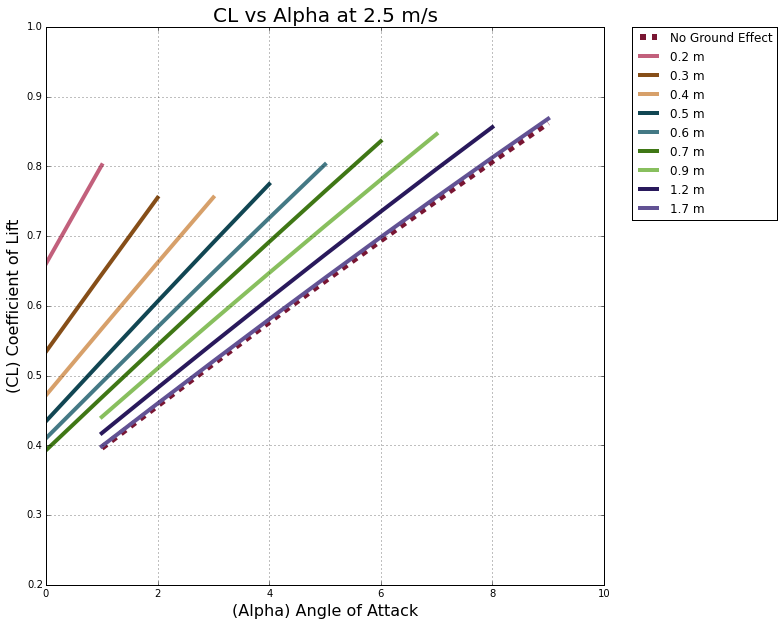Looking at this first study at 2.5 m/s shows the most variation (though most are about the same). I have done the study for angles of attack from 0 to 10, but in reality, the Ekranoplan would never really go above 5, so we could just cut it off and focus on the left side of the graph.

You can see that the no-ground-effect line is the lowest of all curves, next to 1.7 m for height. As stated above in the background information section, the ground effect increases your lift! Pretty drastically of course!

Putting the segmented curves aside, follow along in your head with me. As the craft takes off it will be lowest to the ground experiencing the most ground effect possible, but very quickly it will rise up through the meters (just like planes taking off). So this quick cushion forms and ground effect is present to carry the wings off which is why the lower to the ground, we are seeing the Coefficient of Lift (CL) be much higher. But there is a limitation, and the higher you go, the craft loses the ground effect. According to these simulations, being roughly 1.7 m above the ground resulting in the same effects as the simulation with no ground effect. Makes sense since it's pretty high for a smaller model airplane to be experiencing ground effect.

So looking at this graph, imagine the plane flying in the 2 to 4 angle of attack range (we don't want the tail to hit the water now do we!) and the CL is much much higher at these values vs the non ground effect. Near the tailing edge of 0.4 meters up, that's a CL of about 0.75 compared to the no-ground-effect CL of about 0.52! A massive improvement for no extra cost. This is where the efficiency comes into play. These crafts can float on their cushion, propelling themselves forward with less effort and less fuel costs.

Let's read on for further studies!

In :
#reads of the Airfoil geometries from the cvs data files
alpha00, G00 = numpy.loadtxt('./3ms/00.csv', dtype=float, delimiter=',', unpack=True)
alpha02, G02 = numpy.loadtxt('./3ms/02.csv', dtype=float, delimiter=',', unpack=True)
alpha03, G03 = numpy.loadtxt('./3ms/03.csv', dtype=float, delimiter=',', unpack=True)
alpha04, G04 = numpy.loadtxt('./3ms/04.csv', dtype=float, delimiter=',', unpack=True)
alpha05, G05 = numpy.loadtxt('./3ms/05.csv', dtype=float, delimiter=',', unpack=True)
alpha06, G06 = numpy.loadtxt('./3ms/06.csv', dtype=float, delimiter=',', unpack=True)
alpha07, G07 = numpy.loadtxt('./3ms/07.csv', dtype=float, delimiter=',', unpack=True)
alpha09, G09 = numpy.loadtxt('./3ms/09.csv', dtype=float, delimiter=',', unpack=True)
alpha11, G10 = numpy.loadtxt('./3ms/10.csv', dtype=float, delimiter=',', unpack=True)
alpha16, G16 = numpy.loadtxt('./3ms/16.csv', dtype=float, delimiter=',', unpack=True)

# #lets plot the geometry
%matplotlib inline

#Plotting
size = 10
pyplot.figure(figsize=(size,size))# (y_end-y_start)/(x_end-x_start)*size))
pyplot.grid(True)
pyplot.xlabel('(Alpha) Angle of Attack', fontsize=16)
pyplot.ylabel('(CL) Coefficient of Lift', fontsize=16)
pyplot.title('CL vs Alpha at 3 m/s', fontsize=20)
pyplot.xlim(0, 10.0)
pyplot.ylim(0.2, 1.0)
pyplot.plot(alpha00, G00, label="No Ground Effect",
color=color05, linestyle='--', linewidth=6);
pyplot.plot(alpha02, G02, label="0.2 m",
color=color10, linestyle='-', linewidth=4);
pyplot.plot(alpha03, G03, label="0.3 m",
color=color15, linestyle='-', linewidth=4);
pyplot.plot(alpha04, G04, label="0.4 m",
color=color20, linestyle='-', linewidth=4);
pyplot.plot(alpha05, G05, label="0.5 m",
color=color25, linestyle='-', linewidth=4);
pyplot.plot(alpha06, G06, label="0.6 m",
color=color30, linestyle='-', linewidth=4);
pyplot.plot(alpha07, G07, label="0.7 m",
color=color35, linestyle='-', linewidth=4);
pyplot.plot(alpha09, G09, label="0.9 m",
color=color40, linestyle='-', linewidth=4);
pyplot.plot(alpha12, G10, label="1.1 m",
color=color45, linestyle='-', linewidth=4);
pyplot.plot(alpha17, G16, label="1.6 m",
color=color50, linestyle='-', linewidth=4);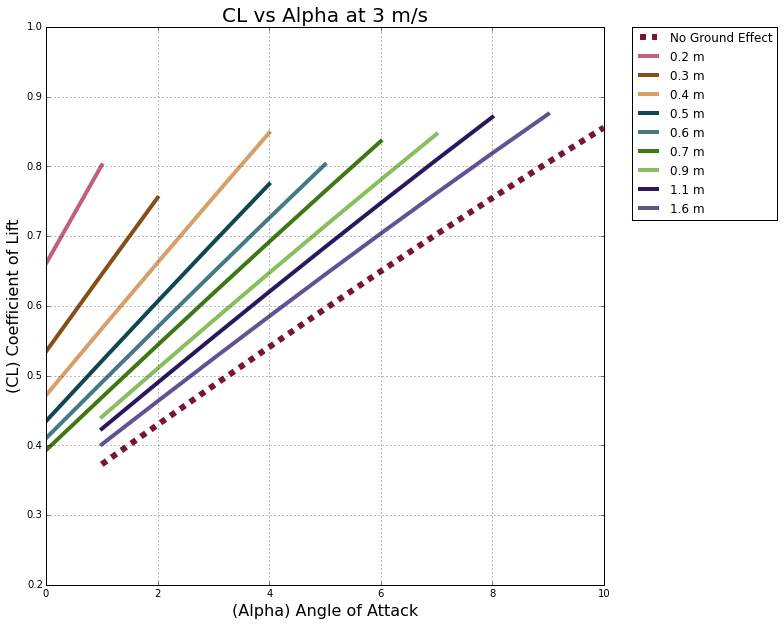Moving at 3 m/s is showing an even further difference as the curves continue. Following that 4 degree angle of attack we are looking at No Ground Effect CL value of 0.55, where the CL of 0.4 m is about 0.85! Huge improvement!

In :
#reads of the Airfoil geometries from the cvs data files
alpha00, G00 = numpy.loadtxt('./10ms/00.csv', dtype=float, delimiter=',', unpack=True)
alpha02, G02 = numpy.loadtxt('./10ms/02.csv', dtype=float, delimiter=',', unpack=True)
alpha03, G03 = numpy.loadtxt('./10ms/03.csv', dtype=float, delimiter=',', unpack=True)
alpha04, G04 = numpy.loadtxt('./10ms/04.csv', dtype=float, delimiter=',', unpack=True)
alpha05, G05 = numpy.loadtxt('./10ms/05.csv', dtype=float, delimiter=',', unpack=True)
alpha06, G06 = numpy.loadtxt('./10ms/06.csv', dtype=float, delimiter=',', unpack=True)
alpha07, G07 = numpy.loadtxt('./10ms/07.csv', dtype=float, delimiter=',', unpack=True)
alpha09, G09 = numpy.loadtxt('./10ms/09.csv', dtype=float, delimiter=',', unpack=True)
alpha12, G12 = numpy.loadtxt('./10ms/12.csv', dtype=float, delimiter=',', unpack=True)
alpha17, G17 = numpy.loadtxt('./10ms/17.csv', dtype=float, delimiter=',', unpack=True)

# #lets plot the geometry
%matplotlib inline

#Plotting
size = 10
pyplot.figure(figsize=(size,size))# (y_end-y_start)/(x_end-x_start)*size))
pyplot.grid(True)
pyplot.xlabel('(Alpha) Angle of Attack', fontsize=16)
pyplot.ylabel('(CL) Coefficient of Lift', fontsize=16)
pyplot.title('CL vs Alpha at 5 m/s', fontsize=20)
pyplot.xlim(0, 10.0)
pyplot.ylim(0.2, 1.0)
pyplot.plot(alpha00, G00, label="No Ground Effect",
color=color05, linestyle='--', linewidth=6);
pyplot.plot(alpha02, G02, label="0.2 m",
color=color10, linestyle='-', linewidth=4);
pyplot.plot(alpha03, G03, label="0.3 m",
color=color15, linestyle='-', linewidth=4);
pyplot.plot(alpha04, G04, label="0.4 m",
color=color20, linestyle='-', linewidth=4);
pyplot.plot(alpha05, G05, label="0.5 m",
color=color25, linestyle='-', linewidth=4);
pyplot.plot(alpha06, G06, label="0.6 m",
color=color30, linestyle='-', linewidth=4);
pyplot.plot(alpha07, G07, label="0.8 m",
color=color35, linestyle='-', linewidth=4);
pyplot.plot(alpha09, G09, label="1.0 m",
color=color40, linestyle='-', linewidth=4);
pyplot.plot(alpha12, G12, label="1.5 m",
color=color45, linestyle='-', linewidth=4);
pyplot.plot(alpha17, G17, label="2.1 m",
color=color50, linestyle='-', linewidth=4);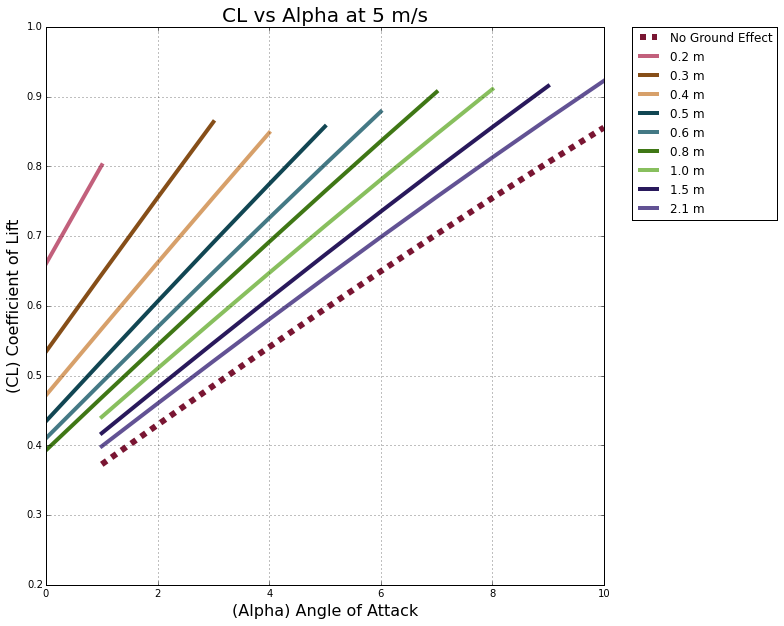In :
#reads of the Airfoil geometries from the cvs data files
alpha00, G00 = numpy.loadtxt('./5ms/00.csv', dtype=float, delimiter=',', unpack=True)
alpha02, G02 = numpy.loadtxt('./5ms/02.csv', dtype=float, delimiter=',', unpack=True)
alpha03, G03 = numpy.loadtxt('./5ms/03.csv', dtype=float, delimiter=',', unpack=True)
alpha04, G04 = numpy.loadtxt('./5ms/04.csv', dtype=float, delimiter=',', unpack=True)
alpha05, G05 = numpy.loadtxt('./5ms/05.csv', dtype=float, delimiter=',', unpack=True)
alpha06, G06 = numpy.loadtxt('./5ms/06.csv', dtype=float, delimiter=',', unpack=True)
alpha08, G08 = numpy.loadtxt('./5ms/08.csv', dtype=float, delimiter=',', unpack=True)
alpha10, G10 = numpy.loadtxt('./5ms/10.csv', dtype=float, delimiter=',', unpack=True)
alpha15, G15 = numpy.loadtxt('./5ms/15.csv', dtype=float, delimiter=',', unpack=True)
alpha21, G21 = numpy.loadtxt('./5ms/21.csv', dtype=float, delimiter=',', unpack=True)

# #lets plot the geometry
%matplotlib inline

#Plotting
size = 10
pyplot.figure(figsize=(size,size))# (y_end-y_start)/(x_end-x_start)*size))
pyplot.grid(True)
pyplot.xlabel('(Alpha) Angle of Attack', fontsize=16)
pyplot.ylabel('(CL) Coefficient of Lift', fontsize=16)
pyplot.title('CL vs Alpha at 10 m/s', fontsize=20)
pyplot.xlim(0, 10.0)
pyplot.ylim(0.2, 1.0)
pyplot.plot(alpha00, G00, label="No Ground Effect",
color=color05, linestyle='--', linewidth=6);
pyplot.plot(alpha02, G02, label="0.2 m",
color=color10, linestyle='-', linewidth=4);
pyplot.plot(alpha03, G03, label="0.3 m",
color=color15, linestyle='-', linewidth=4);
pyplot.plot(alpha04, G04, label="0.4 m",
color=color20, linestyle='-', linewidth=4);
pyplot.plot(alpha05, G05, label="0.5 m",
color=color25, linestyle='-', linewidth=4);
pyplot.plot(alpha06, G06, label="0.6 m",
color=color30, linestyle='-', linewidth=4);
pyplot.plot(alpha08, G08, label="0.8 m",
color=color35, linestyle='-', linewidth=4);
pyplot.plot(alpha10, G10, label="1.0 m",
color=color40, linestyle='-', linewidth=4);
pyplot.plot(alpha15, G15, label="1.5 m",
color=color45, linestyle='-', linewidth=4);
pyplot.plot(alpha21, G21, label="2.1 m",
color=color50, linestyle='-', linewidth=4);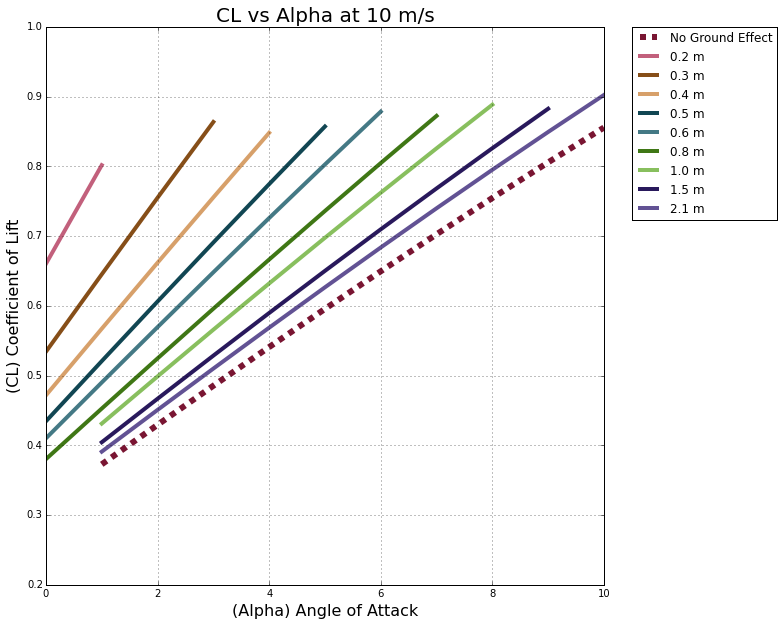It appears not much variation is happening between the 5 m/s and the 10 m/s but there are differences. This again is most likely chalked up to the custom airfoil or not the correct properties for the study or ground effect not being a strong element of XFLR5. But it is a good representation still of what we are trying to study.

So quite intriguing I think, and I understand why the crafts were built for this. Truly a fun concept.

## Plane Studies¶

Now, this is where the fun really began for me, and the headaches. In the program itself, it says adding the different airplane parts were not the best idea for simulation results, but I had to do it anyways. Not only having the custom aircraft imported was fun to see, but to see results was a great feeling too.Simluations run on the entire aircraft were run at 5, 10 and 20 m/s with varied heights and angle of attacks ranging from -5 to 5. I don't believe the plane was designed to fly with angles higher than that, so I limited the range.

In :
#reads of the Airfoil geometries from the cvs data files
alpha00, G00 = numpy.loadtxt('./Full5ms/00.csv', dtype=float, delimiter=',', unpack=True)
alpha05, G05 = numpy.loadtxt('./Full5ms/05.csv', dtype=float, delimiter=',', unpack=True)
alpha06, G06 = numpy.loadtxt('./Full5ms/06.csv', dtype=float, delimiter=',', unpack=True)
alpha07, G07 = numpy.loadtxt('./Full5ms/07.csv', dtype=float, delimiter=',', unpack=True)
alpha08, G08 = numpy.loadtxt('./Full5ms/08.csv', dtype=float, delimiter=',', unpack=True)
alpha09, G09 = numpy.loadtxt('./Full5ms/09.csv', dtype=float, delimiter=',', unpack=True)
alpha10, G10 = numpy.loadtxt('./Full5ms/10.csv', dtype=float, delimiter=',', unpack=True)

# #lets plot the geometry
%matplotlib inline

#Plotting
size = 10
pyplot.figure(figsize=(size,size))# (y_end-y_start)/(x_end-x_start)*size))
pyplot.grid(True)
pyplot.xlabel('(Alpha) Angle of Attack', fontsize=16)
pyplot.ylabel('(CL) Coefficient of Lift', fontsize=16)
pyplot.title('CL vs Alpha at 5 m/s', fontsize=20)
pyplot.xlim(-3.0, 1.5)
pyplot.ylim(0.0, 0.5)
pyplot.plot(alpha00, G00, label="No Ground Effect",
color=color05, linestyle='--', linewidth=6);
pyplot.plot(alpha05, G05, label="0.5 m",
color=color10, linestyle='-', linewidth=4);
pyplot.plot(alpha06, G06, label="0.6 m",
color=color15, linestyle='-', linewidth=4);
pyplot.plot(alpha07, G07, label="0.7 m",
color=color20, linestyle='-', linewidth=4);
pyplot.plot(alpha08, G08, label="0.8 m",
color=color25, linestyle='-', linewidth=4);
pyplot.plot(alpha09, G09, label="0.9 m",
color=color30, linestyle='-', linewidth=4);
pyplot.plot(alpha10, G10, label="1.0 m",
color=color35, linestyle='-', linewidth=4);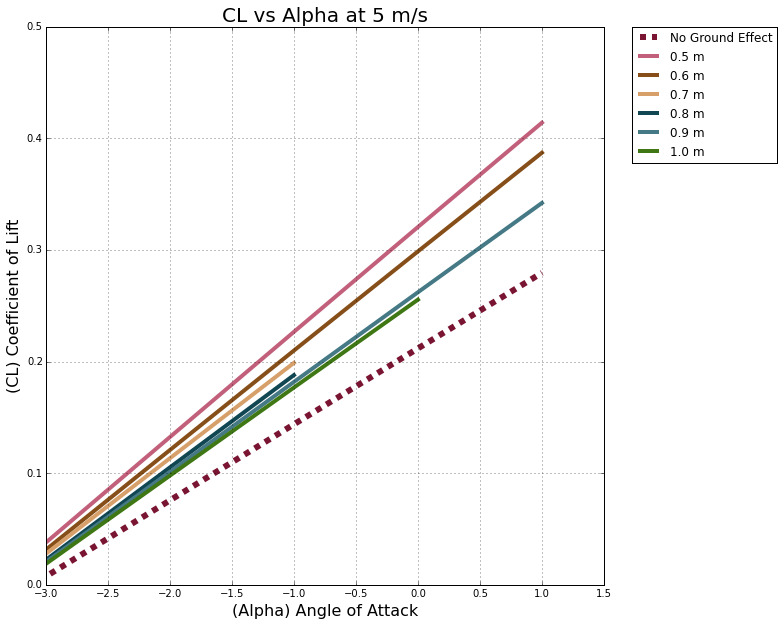By looking at this first graph, one can note that the trend of this project continues through these studies as well. With ground effect, the airplane performs under the same conditions better than without ground effect. In this case, not as large as the airfoil itself, but still an improvement.

In :
#reads of the Airfoil geometries from the cvs data files
alpha00, G00 = numpy.loadtxt('./Full10ms/00.csv', dtype=float, delimiter=',', unpack=True)
alpha04, G04 = numpy.loadtxt('./Full10ms/04.csv', dtype=float, delimiter=',', unpack=True)
alpha05, G05 = numpy.loadtxt('./Full10ms/05.csv', dtype=float, delimiter=',', unpack=True)
alpha06, G06 = numpy.loadtxt('./Full10ms/06.csv', dtype=float, delimiter=',', unpack=True)
alpha07, G07 = numpy.loadtxt('./Full10ms/07.csv', dtype=float, delimiter=',', unpack=True)
alpha08, G08 = numpy.loadtxt('./Full10ms/08.csv', dtype=float, delimiter=',', unpack=True)
alpha09, G09 = numpy.loadtxt('./Full10ms/09.csv', dtype=float, delimiter=',', unpack=True)
alpha10, G10 = numpy.loadtxt('./Full10ms/10.csv', dtype=float, delimiter=',', unpack=True)

# #lets plot the geometry
%matplotlib inline

#Plotting
size = 10
pyplot.figure(figsize=(size,size))# (y_end-y_start)/(x_end-x_start)*size))
pyplot.grid(True)
pyplot.xlabel('(Alpha) Angle of Attack', fontsize=16)
pyplot.ylabel('(CL) Coefficient of Lift', fontsize=16)
pyplot.title('CL vs Alpha at 10 m/s', fontsize=20)
pyplot.xlim(-3.0, 5.0)
pyplot.ylim(0.0, 0.7)
pyplot.plot(alpha00, G00, label="No Ground Effect",
color=color05, linestyle='--', linewidth=6);
pyplot.plot(alpha04, G04, label="0.4 m",
color=color10, linestyle='-', linewidth=4);
pyplot.plot(alpha05, G05, label="0.5 m",
color=color15, linestyle='-', linewidth=4);
pyplot.plot(alpha06, G06, label="0.6 m",
color=color20, linestyle='-', linewidth=4);
pyplot.plot(alpha07, G07, label="0.7 m",
color=color25, linestyle='-', linewidth=4);
pyplot.plot(alpha08, G08, label="0.8 m",
color=color30, linestyle='-', linewidth=4);
pyplot.plot(alpha09, G09, label="0.9 m",
color=color35, linestyle='-', linewidth=4);
pyplot.plot(alpha10, G10, label="1.0 m",
color=color40, linestyle='-', linewidth=4);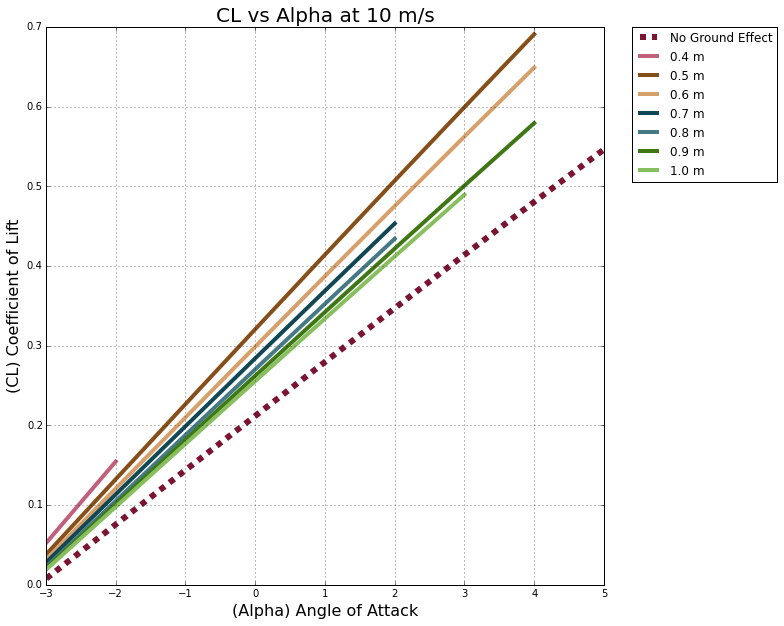In :
#reads of the Airfoil geometries from the cvs data files
alpha00, G00 = numpy.loadtxt('./Full20ms/00.csv', dtype=float, delimiter=',', unpack=True)
alpha04, G04 = numpy.loadtxt('./Full20ms/04.csv', dtype=float, delimiter=',', unpack=True)
alpha05, G05 = numpy.loadtxt('./Full20ms/05.csv', dtype=float, delimiter=',', unpack=True)
alpha06, G06 = numpy.loadtxt('./Full20ms/06.csv', dtype=float, delimiter=',', unpack=True)
alpha07, G07 = numpy.loadtxt('./Full20ms/07.csv', dtype=float, delimiter=',', unpack=True)
alpha08, G08 = numpy.loadtxt('./Full20ms/08.csv', dtype=float, delimiter=',', unpack=True)
alpha09, G09 = numpy.loadtxt('./Full20ms/09.csv', dtype=float, delimiter=',', unpack=True)
alpha10, G10 = numpy.loadtxt('./Full20ms/10.csv', dtype=float, delimiter=',', unpack=True)

# #lets plot the geometry
%matplotlib inline

#Plotting
size = 10
pyplot.figure(figsize=(size,size))# (y_end-y_start)/(x_end-x_start)*size))
pyplot.grid(True)
pyplot.xlabel('(Alpha) Angle of Attack', fontsize=16)
pyplot.ylabel('(CL) Coefficient of Lift', fontsize=16)
pyplot.title('CL vs Alpha at 20 m/s', fontsize=20)
pyplot.xlim(-3.0, 5.5)
pyplot.ylim(0.0, 0.8)
pyplot.plot(alpha00, G00, label="No Ground Effect",
color=color05, linestyle='--', linewidth=6);
pyplot.plot(alpha04, G04, label="0.4 m",
color=color10, linestyle='-', linewidth=4);
pyplot.plot(alpha05, G05, label="0.5 m",
color=color15, linestyle='-', linewidth=4);
pyplot.plot(alpha06, G06, label="0.6 m",
color=color20, linestyle='-', linewidth=4);
pyplot.plot(alpha07, G07, label="0.7 m",
color=color25, linestyle='-', linewidth=4);
pyplot.plot(alpha08, G08, label="0.8 m",
color=color30, linestyle='-', linewidth=4);
pyplot.plot(alpha09, G09, label="0.9 m",
color=color35, linestyle='-', linewidth=4);
pyplot.plot(alpha10, G10, label="1.0 m",
color=color40, linestyle='-', linewidth=4);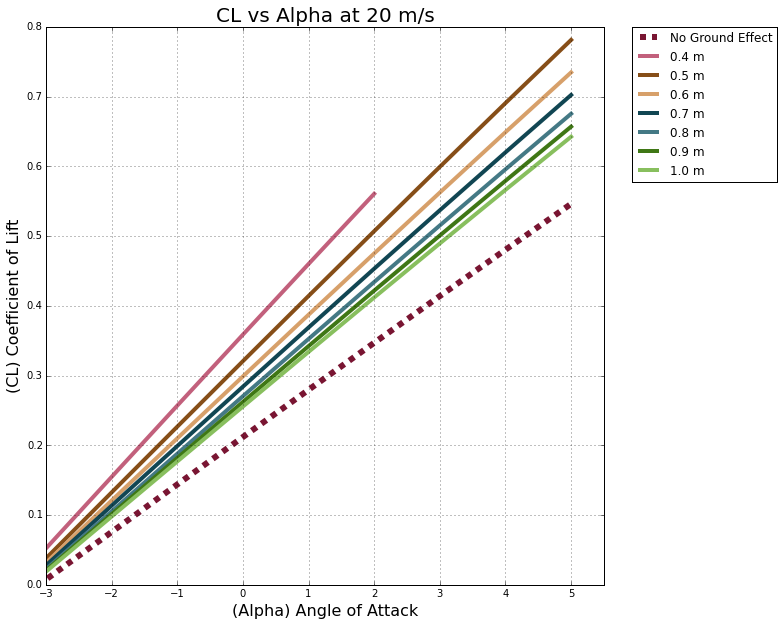The aircraft seems to have some drastic drops in CL vs just the wing itself. This makes sense as the entire body of the aircraft is a drag-inducing entity, especially one that is half a boat on the lower half!

But the simulations still show that ground effect has positive influence with the aircraft in general. Perhaps if one were to add the "Ramming Effect" that the front two engines provide the wings, the CL would be even higher! But that is something that XFLR cannot handle, multi-velocity and multi-physics flows. If someone were to sit down and truly do the fluid mechanics, then that would be quite a challenge. But, I guess at some point, some russian engineer did, as these were actually built and flown!## Conclusion¶

I would like to sum up what this project has accomplished, what I set out to do, and further studies I think would be interesting to keep looking through.

First I would like to say that I believe I have accomplished something I haven't seen before, creating a custom exporter to import custom bodies into XFLR5. This method is a little bit hacky, and will require some "by hand" alterations, but using an open source software, using the simple exporters, and a little creativity, anyone can have some fun creating anything they desire. Hopefully this addition to the online community will yeild some good results!

Second, I have spent the time to do some research into an airplane that I absolutely adore. I wont be chasing any waterfalls here, but I do admire what the USSR had done to finance such a bold project. I still think WIG craft's today have their place, a small niche, but still useful none the less. I was hoping to bring some light into people's lives by doing a study on some ground effect, one which many people have never heard of.

Third, I was looking into learning a new software. A very powerful one at that. With a little toying around, and hopefully some decent inputs, I have achieved some interesting outputs that hopefully has proven the WIG crafts ability to generate lift by just flying close to the ground.

And last, this project was conceived off of an old dream, and the concepts were drawn from a drawing found online. The faithfulness to this from the real thing is something no one can truly prove, as this aircraft was a war machine built in secrecy. Finding the specific data, has been impossible to come by, so hopefully this drawing and these airfoil shapes are true to the real thing. Pictures and models are never the real thing, but hopefully these studies have found something close.

Further studies of course would be to take this data, compare it to someone else's work to truly get some detailed comparison. Or perhaps work more with XFLR5 in hopes to understand the program better, and to get more results of more studies. There are two other main WIG craft designs out there, so perhaps comparison studies also could be done to see the differences in ground effect and their different performances. Many things can be done with these programs and these scripts.

And on that note I say farewell! If you have managed to stick with me through this whole adventure and happen to learn something about a new topic, I am truly honored. If anyone has any questions, or if anyone out in the internet world has questions about what I have done, please use my contact information in the bottom of the page. Label your email as XFLR5 Blender or XFLR5 Custom Airfoil and I will hopefully get back to you as quick as I can!

Cheers,

-George## Vertice Exporter and Body Exporter¶

In [ ]:
#George Teel
#4/20/2015
#
#Super basic python script to export vertices of an object,
#In this case our airfoil shape, and export the locations
#so that XFLR5 can import that shape and then analyze the
#airfoils properly.
#
#This code was figured out from random websites and Blender's wikipedia page.
#

#Import What we need
import bpy

#Sets airfoil mesh object
airfoildata = bpy.data.meshes['Wing']

#Open File
myFile = open('D:/School Documents/AeroHydro/Project/Wing.dat','w')

#Cycles through vertices
for vert in airfoildata.vertices:
myFile.write( '%f %f \n' % (vert.co.x, vert.co.y) )

#Closes File
myFile.close()

In [ ]:
#George Teel
#5/4/2015
#
#Super basic python script to export vertices of an object,
#In this case our body shape, and export the locations
#so that XFLR5 can import that shape and then analyze it
#
#This code was figured out from random websites and Blender's wikipedia page.
#

#Import What we need
import bpy

#Sets airfoil mesh object
bodydata = bpy.data.meshes['BodySimple']

#Open File
myFile = open('D:/School Documents/AeroHydro/Project/EkranoBodySimpledata.dat','w')

#Create the intro Info
myFile.write('Ekranoplan SimpleBody\n')
myFile.write(' \n')
myFile.write('Right Surface Points \n')
myFile.write('        x()        y()          z()\n')
#Cycles through vertices
for vert in bodydata.vertices:
myFile.write( '      %f      %f      %f\n' % (vert.co.x, vert.co.z, vert.co.y) )

#Closes File
myFile.close()

#Still need to modify the data to follow the correct format, but should be easy from there on out.


## Thematic Music¶

https://youtu.be/KxcP7TRY178?t=214

## Contact Info¶

Shoot me an email if you have any questions or would like to discuss further. Please don't forward me any attachments as I won't look at them.

George Teel

Since I hadn't taken any specific information from any one source, most of the A-90 information is found throughout multiple websites. So here is a list of all the websites I found and used for my research, and if any specific websites were used, they have been cited with numbers.

May, James (2008, September 27) Riding the Caspian Sea Monster http://news.bbc.co.uk/2/hi/uk_news/magazine/7638659.stm

 (2014, October 19) CHDB A-90 Orlyonok (Eaglet) (Ekranoplan) Ground Effect Flying Boat / Transport Aircraft (1979) http://www.militaryfactory.com/aircraft/detail.asp?aircraft_id=1251

 (2000, September 07) Project 904/902R Orlan class Amphibious landing craft http://fas.org/man/dod-101/sys/ship/row/rus/904.htm

 Maraev, P., Flying over the waves (WIG "Eaglet") http://www.razlib.ru/transport_i_aviacija/ayerohobbi_1992_02/p3.php

Shechmeister, Matthew (2011, October 06) The Soviet Superplane Program That Rattled Area 51 http://www.wired.com/2011/06/ekranoplan/

Tarantola, Andrew (2013, October 11) This "Caspian Sea Monster" Was a Giant Soviet Spruce Goose http://gizmodo.com/this-caspian-sea-monster-was-a-giant-soviet-spruce-go-1456423681

Ground Effect Vehicle http://en.wikipedia.org/wiki/Ground_effect_vehicle

Random side note, it's pretty peculiar that all these references were done in September or October....

In :
from IPython.core.display import HTML
def css_styling():